## 硬派越野哈弗H9 你身边的“超级英雄”

2018年11月28日 11:55 来源：车行天下 超过：9705次关注

越野的世界总是令人向往的。里面充满着冒险与挑战，他们离开平坦的城市柏油路，离开红绿灯，离开那些呆板的交通指示牌和规矩的斑马线。他们挑战山川、征服高原荒野、穿越沙漠、攀爬虎克之路。他们的世界和胆识、谋略、力量、勇气有关，这是每个男人都希望的生活。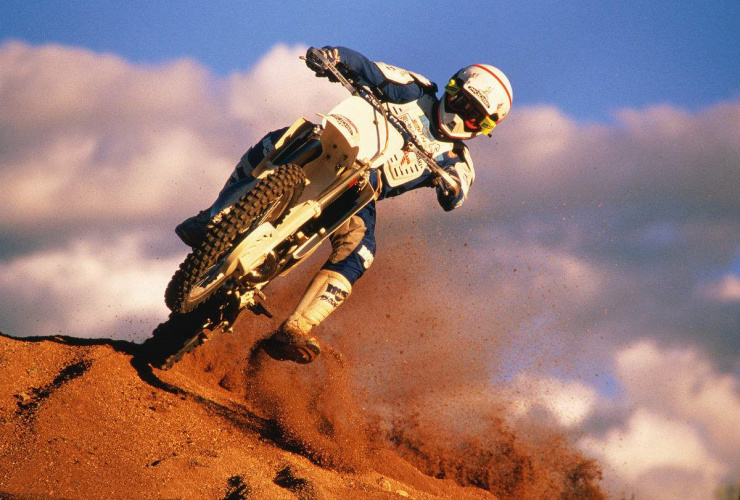工欲善其事必先利其器，想要越野，首选你得购置一台硬派越野车，是越野车而不是SUV。而市面上现行的硬派越野几乎都在50万-300万的价格区间内，这其中有日系代表丰田酷路泽，英系代表路虎卫士，美系代表Jeep牧马人，德系代表奔驰G65。所以说，越野有时候并不仅仅是胆识与勇气的比拼，其中还需要金钱这个硬性指标。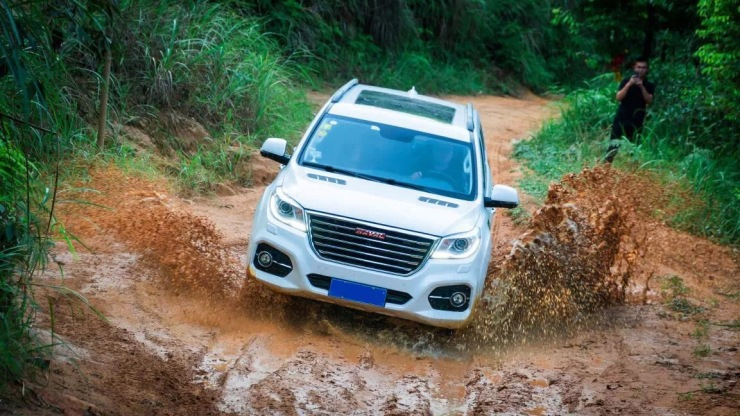当然，凡事既有例外，随着国内车企技术研发实力的成熟，也出现了不少价格实惠，性能优异的硬派越野车，而这其中的佼佼者，便是哈弗旗下的旗舰车型—哈弗H9，它也是中大型SUV市场中唯一的国产硬汉。作为国产硬汉，哈弗H9-8AT搭载了2.0T+8AT的动力总成，其中2.0T双流道汽油发动机，最大功率185kW，最大扭矩355N·m;2.0T双涡轮柴油发动机最大功率140kW，最大扭矩420N·m;拥有非承载式车身，前双叉臂独立悬架、后多连杆非独立悬架，双速TOD智能四驱后桥电子差速锁等等硬派越野车专属构造。此外，哈弗H9的接近角达到28°，离去角达到23°，最小离地间隙更是达到206mm，优异的通过性，让它可以在户外尽情“撒欢”。非常值得一提的还有哈弗H9的全地形控制系统，拥有AUTO、运动、沙地、雪地和泥地等七个模式，其中4L模式能将低扭放大2.48倍，可轻松攀爬60%坡度。驾驶者只需通过中控台的旋钮便能轻松切换七种模式，简单快捷，即便是越野初猎者也能很快上手。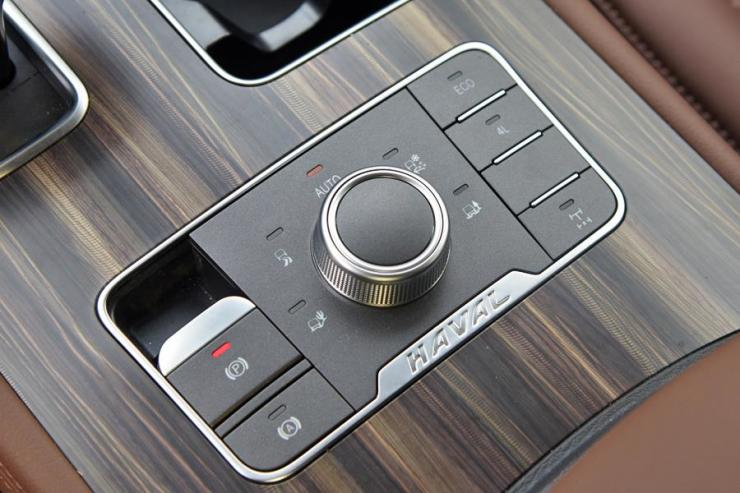也正是这些越野利器，奠定了哈弗H9在自主越野中的领导者地位，今年1-10月，哈弗H9销量达12570辆，累计同比增长23.15%，在中大型SUV市场销量放缓的背景下，显得很是亮眼。哈弗H9能够取得如此优异的市场成绩，与哈弗H9出色的越野性能，可靠的质量品质以及极高的性价比密切相关。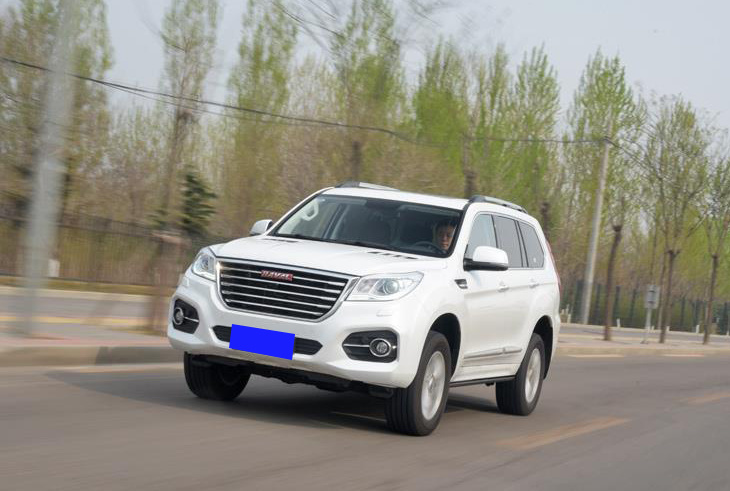当然，更离不开消费者对于这款中大型SUV市场上唯一的国产硬汉，发自内心的喜爱。对于消费者来说，哈弗H9已经不单单只是一款汽车产品，它更像是国产硬派越野高度的象征。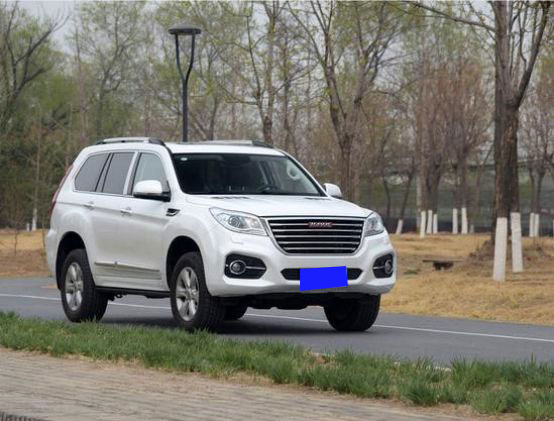这种说法并不是空穴来风，也不是平地拔高。举个例子，在国防领域，曾经国防用车几乎都是丰田陆巡、三菱帕杰罗等车型，而随着自主品牌的兴起与技术的越发成熟，如今，哈弗H9现已被选为国防用车，已经在云南、内蒙古、广西、新疆、天津等其他15个省、自治区及直辖市服役，未来还将继续扩大份额。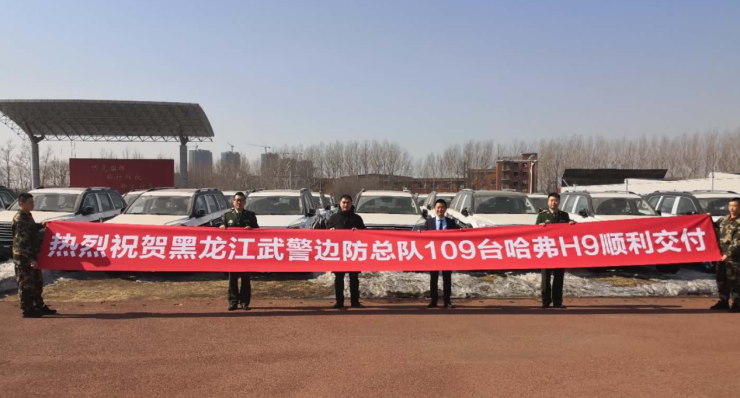当然，国防用车不是儿戏，质量安全与越野性能直接关系到战士的生命安全，而哈弗H9被选入国防用车，也侧面证明了其产品质量与越野性能。事实上，不仅国内，哈弗H9在国外也备受欢迎。日前，柬埔寨和孟加拉国军警系统也都向哈弗采购了大批量哈弗H9作为其军方指定用车。

而在前段时间的俄罗斯车展上，哈弗H9也受到大家的追捧，拥有霸气的外观和全面的机能的哈弗H9对于有着“战斗民族”的俄罗斯民众来说可是巨大的诱惑。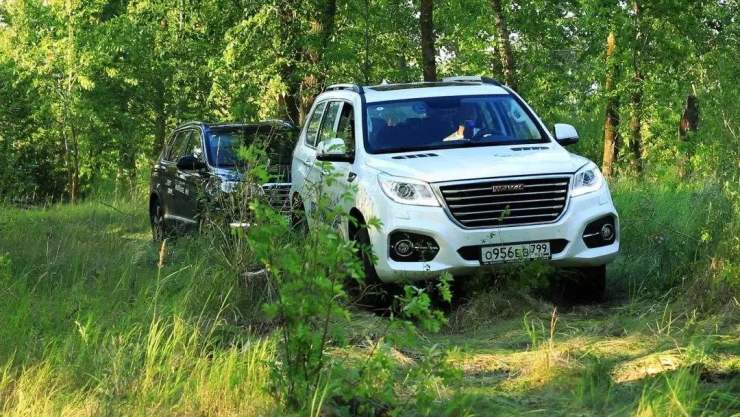不仅于此，哈弗H9更是国内自主品牌首款打破“20万天花板”的车型，在哈弗H9上市以前，自主品牌难有售价突破20万的产品，这部分市场被合资品牌牢牢把控，即便偶尔出现一两辆自主车型，也如昙花一现，消失无踪。而哈弗H9自2014年上市以来，不仅在售价上率先击穿自主20万元的天花板，与合资车型同台竞技，且销量持续上升，获得了众多消费者的认可。可以说，哈弗H9在某种程度上为后来的自主企业树立了信心，其象征地位不言而喻。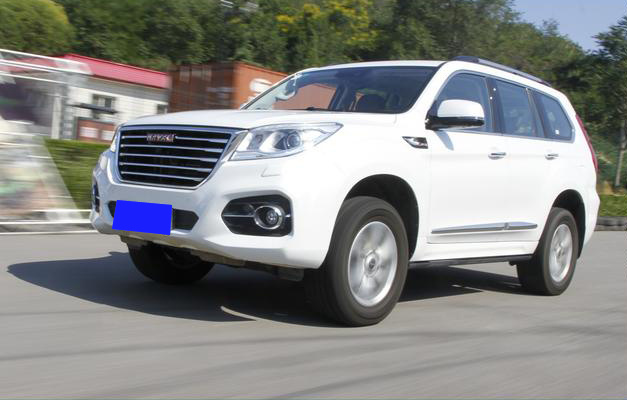写在最后：

哈弗H9在产品质量，越野性能方面都有非常不错表现，并且凭借着较为亲民的价格，更是成为不少越野爱好者的首选车型。但哈弗H9的存在并不仅局限于此，它更像是哈弗在中国越野车领域树立的一面旗帜，如同提及日系越野会联想到陆巡，美系会想到牧马人一样，谈起中国越野，你肯定会联想到哈弗H9。

#### 相关文章

0-500 字已有评论 0条 查看评论>>

﻿
• 快速找车
• 选择品牌
• 选择品牌
• A  奥迪
• A  阿斯顿·马丁
• A  阿尔法·罗密欧
• B  宝沃
• B  布加迪
• B  巴博斯
• B  保时捷
• B  宾利
• B  奔驰
• B  宝马
• B  本田
• B  别克
• B  标致
• B  比亚迪
• B  宝骏
• B  北汽制造
• B  北汽新能源
• B  北汽幻速
• B  北汽威旺
• B  北京汽车
• B  奔腾
• B  北汽绅宝
• C  长安
• C  长安商用
• C  长城
• C  昌河
• D  大众
• D  道奇
• D  DS
• D  东南
• D  东风风神
• D  东风风行
• D  东风小康
• D  东风风度
• D  东风
• F  福特
• F  丰田
• F  菲亚特
• F  法拉利
• F  福田
• F  福迪
• F  福汽启腾
• G  观致
• G  广汽传祺
• G  广汽吉奥
• G  GMC
• H  红旗
• H  汉腾汽车
• H  哈弗
• H  哈飞
• H  海格
• H  海马
• H  华颂
• H  黄海
• H  华泰
• H  恒天
• J  吉利汽车
• J  捷豹
• J  Jeep
• J  江淮
• J  江铃
• J  金杯
• J  九龙
• J  金旅
• K  凯翼
• K  凯迪拉克
• K  克莱斯勒
• K  科尼塞克
• K  卡威
• K  开瑞
• L  路虎
• L  林肯
• L  劳斯莱斯
• L  兰博基尼
• L  雷克萨斯
• L  铃木
• L  雷诺
• L  理念
• L  力帆
• L  莲花汽车
• L  猎豹
• L  路特斯
• L  陆风
• M  马自达
• M  MG
• M  MINI
• M  玛莎拉蒂
• M  摩根
• M  迈凯轮
• N  纳智捷
• O  欧宝
• O  讴歌
• O  欧朗
• Q  奇瑞
• Q  起亚
• Q  启辰
• R  日产
• R  荣威
• R  瑞麒
• S  三菱
• S  斯威汽车
• S  萨博
• S  smart
• S  斯柯达
• S  斯巴鲁
• S  思铭
• S  双龙
• S  上汽大通
• S  双环
• T  特斯拉
• T  腾势
• W  沃尔沃
• W  五菱汽车
• W  五十铃
• W  威兹曼
• W  威麟
• X  现代
• X  雪佛兰
• X  雪铁龙
• X  西雅特
• Y  一汽
• Y  英菲尼迪
• Y  英致
• Y  依维柯
• Y  野马汽车
• Y  永源
• Z  众泰
• Z  中华
• Z  中兴
• Z  知豆
• 选择车系
• 选择车系
• 车型对比
• 选择品牌
• 选择品牌
• A  奥迪
• A  阿斯顿·马丁
• A  阿尔法·罗密欧
• B  宝沃
• B  布加迪
• B  巴博斯
• B  保时捷
• B  宾利
• B  奔驰
• B  宝马
• B  本田
• B  别克
• B  标致
• B  比亚迪
• B  宝骏
• B  北汽制造
• B  北汽新能源
• B  北汽幻速
• B  北汽威旺
• B  北京汽车
• B  奔腾
• B  北汽绅宝
• C  长安
• C  长安商用
• C  长城
• C  昌河
• D  大众
• D  道奇
• D  DS
• D  东南
• D  东风风神
• D  东风风行
• D  东风小康
• D  东风风度
• D  东风
• F  福特
• F  丰田
• F  菲亚特
• F  法拉利
• F  福田
• F  福迪
• F  福汽启腾
• G  观致
• G  广汽传祺
• G  广汽吉奥
• G  GMC
• H  红旗
• H  汉腾汽车
• H  哈弗
• H  哈飞
• H  海格
• H  海马
• H  华颂
• H  黄海
• H  华泰
• H  恒天
• J  吉利汽车
• J  捷豹
• J  Jeep
• J  江淮
• J  江铃
• J  金杯
• J  九龙
• J  金旅
• K  凯翼
• K  凯迪拉克
• K  克莱斯勒
• K  科尼塞克
• K  卡威
• K  开瑞
• L  路虎
• L  林肯
• L  劳斯莱斯
• L  兰博基尼
• L  雷克萨斯
• L  铃木
• L  雷诺
• L  理念
• L  力帆
• L  莲花汽车
• L  猎豹
• L  路特斯
• L  陆风
• M  马自达
• M  MG
• M  MINI
• M  玛莎拉蒂
• M  摩根
• M  迈凯轮
• N  纳智捷
• O  欧宝
• O  讴歌
• O  欧朗
• Q  奇瑞
• Q  起亚
• Q  启辰
• R  日产
• R  荣威
• R  瑞麒
• S  三菱
• S  斯威汽车
• S  萨博
• S  smart
• S  斯柯达
• S  斯巴鲁
• S  思铭
• S  双龙
• S  上汽大通
• S  双环
• T  特斯拉
• T  腾势
• W  沃尔沃
• W  五菱汽车
• W  五十铃
• W  威兹曼
• W  威麟
• X  现代
• X  雪佛兰
• X  雪铁龙
• X  西雅特
• Y  一汽
• Y  英菲尼迪
• Y  英致
• Y  依维柯
• Y  野马汽车
• Y  永源
• Z  众泰
• Z  中华
• Z  中兴
• Z  知豆
• 选择车系
• 选择车系
• 选择车型
• 选择车型
• 意见反馈